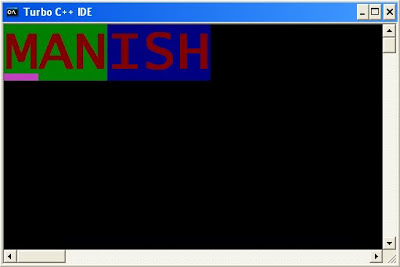## Working with video memory using far pointers

### Working with video memory using far pointers

VERY INTERESTING THING:

Video memory

Segment number 0XA and 0XB is known as video memory. There are two types of video memory.
(a) Text video memory
(b) Graphics video memory

(a) Text video memory:

Segment number 0XB is known as text video memory. This segment is divided into 25 rows and 80 columns which creates 80*25=2000 cells.
Size of each cell is two byte. Each cell is divided into two parts each of size one byte.

(a) Text byte: First byte stores character information. It stores character as ASCII code.

(b) Color byte: Second byte stores color information of text byte character.
In other word we can say each even byte stores character and each odd byte stores color.

Simple example:

(q)What will be output of following c program?

#include<stdio.h>

int main(){

int i;
char far *ptr=(char *)0XB8000000;

*ptr='A';
*(ptr+1)=4;

return 0;
}

Output: It will display character A in the red color as shown following screen dump:Color scheme:Color byte of size 8 bit stores the color information in the following manner.

First four bits stores color information of character.

0000 0001: Blue color (1)
0000 0010: Green color (2)
0000 0100: Red color (4)
0000 1000: To increase the intensity of color. (8)

Note: Any other number will generate mixture of above four basic colors.
Next four bits stores color information of background of character.

0001 0000: Blue color (16)
0010 0000: Green color (32)
0100 0000: Red color (64)
1000 0000: To increase the intensity of color. (128)

Note: Any other number will generate after mixing of above four basic colors.

Examples:

(1)What will be output of following c program?

#include<stdio.h>

int main(){

int i;
char far *ptr=(char *)0XB8000000;

*ptr='A';
*(ptr+1)=1;

*(ptr+2)='B';
*(ptr+3)=2;

*(ptr+4)='C';
*(ptr+5)=4;

return 0;
}

Output:(2)What will be output of following c program?

#include<stdio.h>

int main(){

int i;
char far *ptr=(char *)0XB8000000;

*ptr='W';
*(ptr+1)=1;

*(ptr+2)='O';
*(ptr+3)=2;

*(ptr+4)='R';
*(ptr+5)=4;

*(ptr+6)='L';
*(ptr+7)=1;

*(ptr+8)='D';
*(ptr+9)=2;

return 0;
}

Output:(3)What will be output of following c program?

#include<stdio.h>

//Mixture of basic color
int main(){

int i;
char far *ptr=(char *)0XB8000000;

*ptr='W';
*(ptr+1)=3;

*(ptr+2)='O';
*(ptr+3)=5;

*(ptr+4)='R';
*(ptr+5)=6;

*(ptr+6)='L';
*(ptr+7)=7;

*(ptr+8)='D';
*(ptr+9)=3;

return 0;
}

Output:(4)What will be output of following c program?

#include<stdio.h>

//To increase the intensity of color.
int main(){

int i;
char far *ptr=(char *)0XB8000000;

*ptr='P';
*(ptr+1)=1+8;

*(ptr+2)='O';
*(ptr+3)=2+8;

*(ptr+4)='I';
*(ptr+5)=3+8;

*(ptr+6)='N';
*(ptr+7)=4+8;

*(ptr+8)='T';
*(ptr+9)=5+8;

*(ptr+10)='E';
*(ptr+11)=6+8;

*(ptr+12)='R';
*(ptr+13)=7+8;

return 0;
}

Output:(5)What will be output of following c program?

#include<stdio.h>

// for background color
int main(){

int i;
char far *ptr=(char *)0XB8000000;

*ptr='M';
*(ptr+1)=4+32;

*(ptr+2)='A';
*(ptr+3)=4+32;

*(ptr+4)='N';
*(ptr+5)=4+32;

*(ptr+6)='I';
*(ptr+7)=4+16;

*(ptr+8)='S';
*(ptr+9)=4+16;

*(ptr+10)='H';
*(ptr+11)=4+16;

return 0;
}

Output:Anonymous
23 April 2014 at 01:31

Nice Blog and Great article…its always working for me well…Keep going !!
prestigeLakeside Habitat ,
prestigeLakeside Habitat and
prestigeLakeside HabitatAnonymous
23 April 2014 at 01:32

Nice Blog and Great article…its always working for me well…Keep going !!
prestigeLakeside Habitat ,
prestigeLakeside Habitat and
prestigeLakeside HabitatAnonymous
23 April 2014 at 01:32Anonymous
23 April 2014 at 01:32

This nice and useful blog....Thanks for this post, Thanks for posting this informative blog...well done.

prestige sunrise park
prestige sunrise park and
prestige sunrise park bangaloreAnonymous
23 April 2014 at 01:32Anonymous
23 April 2014 at 01:33

Thanks for posting this informative blog...well done
Prestige Falcon city
Prestige Falcon city bangaloreAnonymous
23 April 2014 at 01:33

Thanks for sharing the useful information with us..Keep going
purvakara westend
purvakara westend bangaloreAnonymous
23 April 2014 at 01:33

I am glad to read this post, its an interesting one. I am always searching for quality posts and articles

purva palm beach

Purva palm beachAnonymous
23 April 2014 at 01:33

This nice and helpful blog....Thanks for this post, Thanks for posting this instructive blog...well done.

prestige royal gardens , prestige royal gardens bangalore, and prestige royale gardensAnonymous
23 April 2014 at 01:33Anonymous
23 April 2014 at 01:34

Thanks for this post, Thanks for posting this informative blog.....well done.

Mjr pearl
Mjr pearl
Mjr pearlAnonymous
23 April 2014 at 01:34Anonymous
23 April 2014 at 01:34

Excellent blog and high quality article...........

Purva midtown
Purva midtown bangalore
Purva midtown